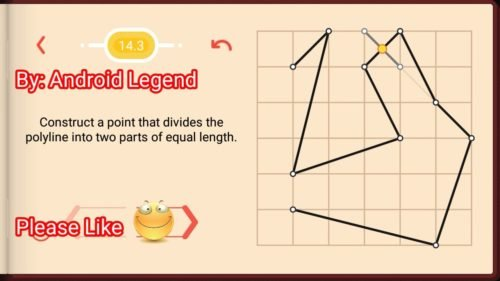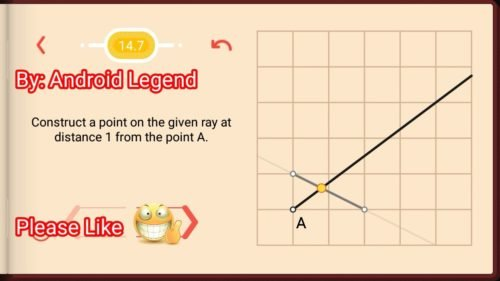# Pythagorea Level 14.1 14.2 14.3 14.4 14.5 14.6 14.7 14.8 14.9 Solution/AnswersPythagorea is android/iOS app developed by Horis International Limited. Solutions hints and answers to pythagorea are available in this post scroll down to find solutions to all the levels.

This game is mostly focused on geometric puzzles and construction. the workspace is divided into grids to draw lines. You should know all the basic Math operations. All lines and shapes are drawn on a grid whose cells are squares. Most of the game levels can be answered using natural intuition and bye some basic laws of geometry.

You have to connect points on the grid using straight lines to construct an element, you can even use intersection points to draw. some levels are very easy some are of medium difficulty and some are very hard to solve, that’s why I am providing solutions to all the problems.

If you have forgotten basic course you did in your elementary education, this game is for you to revise all the concept using a single game or if you don’t know any of the geometry this game features “i” button from where you can learn about all the shapes and geometry and then play this game to enhance your geometry skill.And do not miss your chance to familiarize children with mathematics. Pythagorea is an excellent way to make friends with geometry and benefit from spending time together.

If you are here for levels other than ‘Length and Proportions’ Go to directory of all other levels at : http://www.puzzlegamemaster.com/pythagorea-all-answers-solutions/

• Pythagorea Level 14.1: Construct a point on the segment that divides it in the ratio 2:3.• Pythagorea Level 14.2: Construct a point that divides the polyline into two parts of equal length.• Pythagorea Level 14.3: Construct a point that divides the polyline into two parts of equal length.• Pythagorea Level 14.4: Construct a point that divides the polyline into two parts of equal length.• Pythagorea Level 14.5: Construct a point on the segment that divides it in the ratio 2:3.• Pythagorea Level 14.6: Construct two points that divide the segment into three equal parts.• Pythagorea Level 14.7: Construct a point on the given ray at distance 1 from the point A.• Pythagorea Level 14.8: Construct the midpoint of the segment
AB.• Pythagorea Level 14.9: Construct the midpoint of the segment
AB.Feel free to comment below if you have any doubts regarding the solutions. I will try to help you guys. All other levels are posted on this blog please visit them too.

### 8 thoughts on “Pythagorea Length and Proportions All Levels (14.1-14.9) Solutions/Answers”

•August 26, 2019 at 8:34 pm

What kind of bullshit is this? Why you put the points and lines in 14.8 and 14.9 where you put it? What is this shit useful for? I dont want to know the solution or the steps but the reason why. This guy is losing his time for nothing, completely stupid.

•March 18, 2019 at 3:25 pm

I’m having a hard time seeing why the solutions for 14.7-9 work, can someone explain the principals behind it?

•December 7, 2018 at 10:59 am

14.1 and 14.5 are wrong!
The question is 2:3 but the only answer accepted is 2:5 🙁
I think in 14.1 should be 2:3 and programmer correct the answer accepted to 2:3 and not 2:5 as it is at this point, and 14.5 should change the question to 2:5 as it should be more difficult…
Anyway a very good game!!!

•October 30, 2018 at 9:40 pm

I understand every example except 14.5, I don’t get why it works.

•February 27, 2018 at 5:33 pm

14.1: I really can’t see thisntonbe correct. Measure it with a ruler. I think the accepted solution is incorrect! Please comment!

The point accepted is close to the 0.5 point than the 0.75 point.

•February 27, 2018 at 8:18 pm

the point divides the 4 unit line into 1.6 units and 2.4 units, Hence giving ratio 2:3,
You can see those two constructed parallel lines are divided into 2:3 ratio, hence given line must also be divided into ratio of 2:3

•July 19, 2018 at 11:36 pm
•